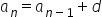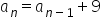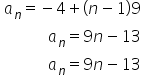Maths-
General
Easy

Question

# Write recursive formula and explicit formula. -4, 5,14,23,32,...

## The correct answer is: a_n=9n-13.

### Step 1 of 2:We have given a sequence -4,5,14,23,32,...The given sequence is an AP.We know that the recursive formula for any AP is, where d is common difference.Here the common difference is 9So, The recursive formula isStep 2 of 2:The given sequence is an AP.So, The explicit formula will be.#### With Turito Foundation.#### Get an Expert Advice From Turito.